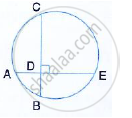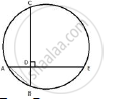Share

In the Given Figure, Ae and Bc Intersect Each Other as Point D. If ∠Cde = 90°, Ab = 5cm, Bd = 4cm and Cd = 9 Cm Find Ae. - ICSE Class 10 - Mathematics

ConceptArc and Chord Properties - If Two Chords Intersect Internally Or Externally Then the Product of the Lengths of the Segments Are Equal

Question

In the given figure, AE and BC intersect each other as point D.If ∠CDE = 90°, AB = 5cm, BD = 4cm and CD = 9 cm find AE.

SolutionAD = sqrt(AB^2 - DB^2) =  sqrt(5^2 - 4^2) =  sqrt(25- 16) = sqrt(9) = 3cm
Now, since the two chords AE and BC intersect at D,
AD × DE = CD × DB
3 × DE = 9 × 4

DE = (9xx4)/3 =12
Hence, AE = AD + DE = (3 + 12) = 15 cm

Is there an error in this question or solution?

APPEARS IN

Solution In the Given Figure, Ae and Bc Intersect Each Other as Point D. If ∠Cde = 90°, Ab = 5cm, Bd = 4cm and Cd = 9 Cm Find Ae. Concept: Arc and Chord Properties - If Two Chords Intersect Internally Or Externally Then the Product of the Lengths of the Segments Are Equal.
S